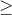# 1 suppose you are given a row vector 39 a 39 of size 1 x n where n 1 note that n 1 i 5346851

1. Suppose you are given a row vector &#39;a&#39; of size 1 x n where n1. Note that n = 1 is possible. Write a function

function [b] = Avg_nbd (a)

………….

end

in which every element of &#39;b&#39; is the average of every element of &#39;a&#39; and its immediate neighbors. For example, the function should work as follow:

>> Avg_nbd (  )

ans =

2

>> Avg_nbd ( [1,1,1] )

ans =

1    1    1

>> a = [0, 1, 1, 1, 0];

>> Avg_nbd ( a )

ans =

0.5000      0.6667      1.0000      0.6667      0.5000

2. Now, generalize your function Avg_nbd s0 that input &#39;A&#39; is any 3 x n matrix. For example:

>> A = [1; 1; 1];

>> Avg_nbd ( A )

ans =

1

1

1

>> A = [1, 0; 1, 2; 1, 0];

>> Avg_nbd ( A )

ans =

1.0000      1.0000

0.8333      0.8333

1.0000      1.0000

>> A = [1, 1, 1; 1, 2, 3; 1, 3, 6];

>> Avg_nbd ( A )

ans =

1.2500      1.5000      1.7500

1.5000      2.1111       2.6667

1.7500      2.6667      3.5000

3. Now, generalize your code so that input &#39;A&#39; is any n x n matrix. For example:

>> A

A =

1          1          1          1          1

1          2          3          1          1

1          3          6          1          1

8          1          6          2          2

3          5          7          2          2

4          9          2          2          2

>>Avg_nbd ( A )

ans =

1.2500       1.5000    1.5000     1.3333       1.0000

1.5000       2.1111        2.1111        1.7778       1.0000

2.6667       3.4444       2.7778        2.5556       1.3333

3.5000       4.4444       3.6667        3.2222       1.6667

5.0000       5.0000       4.0000        3.0000       2.0000

5.2500       5.0000       4.5000        2.8333       2.0000

Please provide the complete MATLAB code! Make sure to taste with all these example! You can provide only one code that can be used with all 3 different type mentioned above.